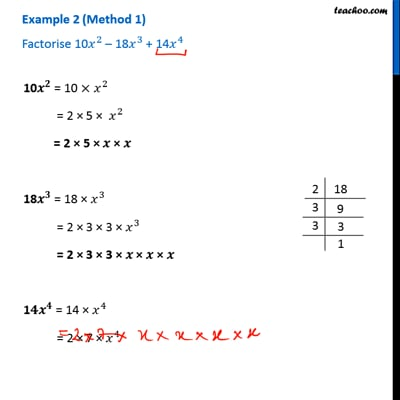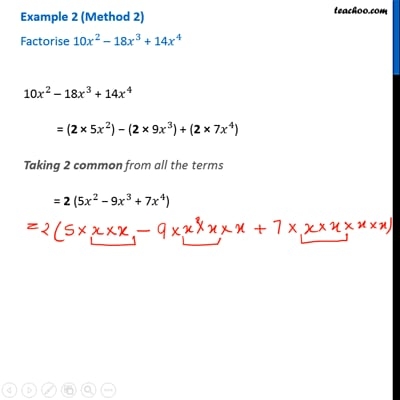Factorisation using common factors

Chapter 12 Class 8 Factorisation
Concept wiseThis video is only available for Teachoo black usersThis video is only available for Teachoo black users

Get live Maths 1-on-1 Classs - Class 6 to 12

### Transcript

Example 2 (Method 1) Factorise 10𝑥^2 – 18𝑥^3 + 14𝑥^4 10𝑥^2 = 10 × 𝑥^2 = 2 × 5 × 𝑥^2 = 2 × 5 × 𝑥 × 𝑥 18𝑥^3 = 18 × 𝑥^3 = 2 × 3 × 3 × 𝑥^3 = 2 × 3 × 3 × 𝑥 × 𝑥 × 𝑥 14𝑥^4 = 14 × 𝑥^4 = 2 × 7 × 𝑥^4 = 2 × 7 × 𝑥 × 𝑥 × 𝑥 × 𝑥 Now, 10𝑥^2 = 2 × 5 × 𝑥 × 𝑥 18𝑥^3 = 2 × 3 × 3 × 𝑥 × 𝑥 × 𝑥 14𝑥^2 = 2 × 7 × 𝑥 × 𝑥 × 𝑥 × 𝑥 So, 2, 𝑥, 𝑥 are common factors. 10𝑥^2 + 18𝑥^3+14𝑥^4 = (2 × 5 × 𝑥 × 𝑥) − (2 × 3 × 3 × 𝑥 × 𝑥" × " 𝑥) + (2 × 7 × 𝑥 × 𝑥 × 𝑥 × 𝑥) = 2 × 𝑥 × 𝑥 (5 − (3 × 3 × 𝑥) + (7 × 𝑥 × 𝑥)) = 2𝑥^2 (5 − 9𝑥 + 7𝑥^2) = 2𝒙^𝟐 (7𝒙^𝟐 − 9𝒙 + 5) Example 2 (Method 2) Factorise 10𝑥^2 – 18𝑥^3 + 14𝑥^410𝑥^2 – 18𝑥^3 + 14𝑥^4 = (2 × 5𝑥^2) − (2 × 9𝑥^3) + (2 × 7𝑥^4) Taking 2 common from all the terms = 2 (5𝑥^2 − 9𝑥^3 + 7𝑥^4) = 2 ( (5 × 𝑥^2) − (9𝑥 × 𝑥^2) + (7𝑥^2 × 𝑥^2) ) Taking 𝑥^2 common from all the terms = 2𝑥^2 (5 − 9𝑥 + 7𝑥^2) = 2𝒙^𝟐 (7𝒙^𝟐 − 9𝒙 + 5)